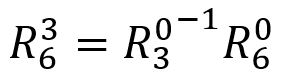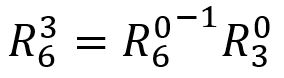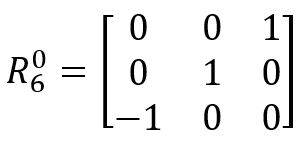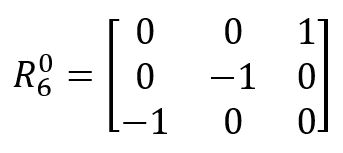Robotics 2
Inverse Kinematics for Rotation
QuizQuestion 3:  Shown here is a rotation matrix.  Is it a valid rotation matrix?
Question 7:  Suppose that I have attached a gripper to the manipulator end-effector with the tines (the pointy part) pointing in the X6 direction.  I want to pick up something on the floor, so that the tines are pointing in the -Z0 direction.  Which of these rotation matrices might I want for the rotation from frame 0 to frame 6?
Questions 8-10:  I would like the complete rotation matrix from the base frame to the end-effector frame to be as shown here. What values should I set for Theta 4, 5, and 6?  Answer in units of radians, and round to three decimal places.

Hint 1: Use your Python code to solve this.
Hint 2: This is an especially tricky question, because your first solution will probably make the R3_6_Check matrix NOT MATCH the R3_6 matrix.  You will have to pick one of the other possible solutions for one of the theta angles by adding pi to it.  When you have the right solution, your R0_6 will match the one given here, AND your R3_6_Check matrix will match your R3_6 matrix.
Question 2: What are the two rules a matrix must follow in order to be considered a valid rotation matrix?
Question 1:  In order to use the method in the video to do inverse kinematics for a manipulator with more than 3 joints, we have to make an important assumption.  What is that assumption?
The manipulator consists of an 'arm' of three joints with a spherical wrist attached to the end
The first three joints of the manipulator are one of our standard manipulator types
The first three joints are entirely responsible for positioning the end-effector, and any additional joints are responsible for the orientation
The manipulator is not kinematically-redundant
The vector length of every row and every column must be equal to 1, and the matrix must describe a right-hand coordinate system
Each row and each column can only have one non-zero value in it, and the matrix must be invertible
Every row and every column must have a vector length of 0, and the matrix must have 3 rows and 3 columns
The matrix must be invertible, and the matrix must have 3 rows and 3 columns
Yes
NoQuestion 4:  Shown here is a rotation matrix.  Is it a valid rotation matrix?
Yes
NoNo
Yes
Question 5:  Shown here is a rotation matrix.  Is it a valid rotation matrix?Question 6:  Which of these equations tells how to solve for the rotation matrix from frame 3 to frame 6 when I already know the rotation matrix from frame 0 to frame 3 and the rotation matrix from frame 0 to frame 6?Theta 5 =
Theta 6 =
Theta 4 =